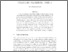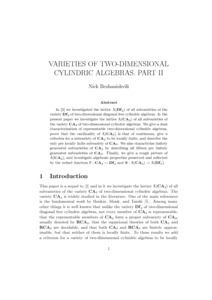# PP-2002-18: Varieties of Two-Dimensional Cylindric Algebras. Part II

PP-2002-18: Bezhanishvili, Nick (2002) Varieties of Two-Dimensional Cylindric Algebras. Part II. [Report]Preview Text (Full Text (PDF)) PP-2002-18.text.pdf Download (267kB) | PreviewText (Full Text (PS)) PP-2002-18.text.ps.gz Download (263kB)Text (Abstract) PP-2002-18.abstract.txt Download (1kB)

## Abstract

In the precursor to this report, we investigated the lattice
$\Lambda(Df_2)$ of all subvarieties of the variety $Df_2$ of
two-dimensional diagonal free cylindric algebras. In the present paper
we investigate the lattice $\Lambda(CA_2)$ of all subvarieties of the
variety $CA_2$ of two-dimensional cylindric algebras. We give a dual
characterization of representable two-dimensional cylindric algebras,
prove that the cardinality of $\Lambda(CA_2)$ is that of continuum,
give a criterion for a subvariety of $CA_2$ to be locally finite, and
describe the only pre locally finite subvariety of $CA_2$. We also
characterize finitely generated subvarieties of $CA_2$ by describing
all fifteen pre finitely generated subvarieties of $CA_2$. Finally, we
give a rough picture of $\Lambda(CA_2)$, and investigate algebraic
properties preserved and reflected by the reduct functors
$F : CA_2 \to Df_2$ and $\Phi : \Lamda(CA_2) \to \Lambda(Df2)$.

Item Type: Report PP-2002-18 Prepublication (PP) Series 2002 12 Oct 2016 14:36 12 Oct 2016 14:36 https://eprints.illc.uva.nl/id/eprint/82View Item